### Home > CALC > Chapter 12 > Lesson 12.1.4 > Problem12-51

12-51.
1. Integrate each function. Homework Help ✎

1.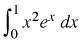2.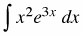3.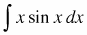4.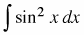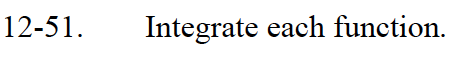All of these integrals require the use of integration by parts.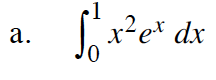Note: The following steps ignore the bounds of integration. Be sure to apply them at the end.
Let f = x2 and dg = exdx.
Then df = 2x dx and g = ex.

For the integral:
Let f = 2x and dg = exdx.
Then df = 2dx and g = ex.

You can now integrate the integral that is left without using integration by parts.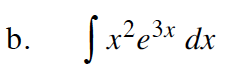Use the same process outlined in part (a), but use e3x instead of ex.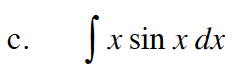Let f = x and dg = sin(x).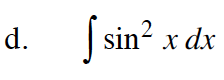Let f = sin(x) and dg = sin(x)dx.

Now solve this equation for the original integral.